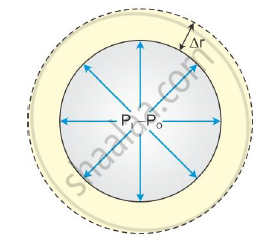Share

# Derive an Expression for Excess Pressure Inside a Drop of Liquid. - Physics

#### Question

Derive an expression for excess pressure inside a drop of liquid.

#### Solution

Consider a liquid drop of radius R. Due to the surface tension, the molecules lying on the surface of the liquid drop will experience a resultant force inwards, perpendicular to the surface. The pressure inside the liquid drop must be greater than the pressure outside as the size of the drop cannot be reduced to zero. This excess pressure inside the drop provides a force outwards, perpendicular to the surface to counter balance the resultant force due to the surface tension.Let the pressure outside the liquid drop be Po and the inside pressure be Pi.

The excess pressure inside the drop = (Pi − Po).

Let T be the surface tension of the liquid.

Let the radius of the drop increase from r to (r + Δr) due to excess pressure.

The work done by the excess pressure is given by

dW = Force x Displacement

= (Excess pressure x Area) x (Increase in radius)

= [(Pi - Po) x 4πr2] x Δr                                      .........(1)

Let the initial surface area of the drop be A1 = 4πr2.

The final surface area of the drop is A2 = 4π(r+Δr)2.

A2 = 4π(r2 + 2rΔr + Δr2)

= 4πr2 + 8πrΔr +4πΔr2

As Δr is very small, Δr2 can be neglected, i.e. 4πΔr2 ≅ 0

Therefore,

A2 = 4πr2 + 8πrΔr

Therefore, increase in the surface area of the drop dA = A2 − A1

= 4πr2 + 8πrΔr − 4πr2

= 8πrΔr

Work done to increase the surface area by 8πrΔr is the extra surface energy.

dW = T × d A

where T is the surface energy

dW = T × 8πrΔr                                                         ..........(2)

Comparing (1) and (2), we get

[(Pi - Po) x 4πr2] x Δr = T x 8πrΔr

Pi - Po = 2T/r

The above equation gives the excess pressure inside a liquid drop.

Is there an error in this question or solution?